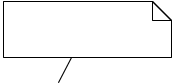# Introduction to algorithms and UML

#### What is an algorithm?

The simplest way to describe a purpose of an algorithm is to say is that it’s a step-by-step guide to do something. Algorithms may be used to describe the behavior of real world objects and processes e.g. how to tie shoelaces, wash your hair or make an omelet. However most commonly algorithms can be found in the fields of mathematics and computer science. Some of the things that can be described with an algorithm include searching, finding the lowest or the highest values, sorting, converting between numeral systems (radix) etc.

The most important thing about an algorithm is that it successfully completes the task at hand. However since things can be done in numerous ways, the next on the list becomes how effective it is – how much time it takes to solve a problem? How much does the time increase when the problem increases in size (e.g. how does the time taken increase when going from sorting 10 digits to 10 billion digits?)

During the course we’ll cover some simpler algorithms, how to present them in pseudocode and using modelling tools.

An algorithm must be language independent – You should be able to use the same algorithm in a variety of languages, meaning you can’t use any specific code within the algorithm itself.

#### What is UML?

UML (universal modeling language), as the name stands, is used for modelling various and in fact, quite a large variety of things. We’ll introduce you to activity diagrams, which is only a small fraction of what UML is capable of. This will be used to visualize algorithms and processes that the programs we create can and will do. Other types of algorithms may be covered in various courses in the future or past.

#### Shapes used

Symbol DESCRIPTIONInitial nodeEnd nodeActionDecision node

Join nodeCommentFork node (for parallel activities)
Activities must not depend on each other!Join node (for parallel activities)

#### ArgoUML

###### Creating a new activity diagram###### Exporting graphics from UML (for your documentation)